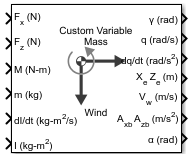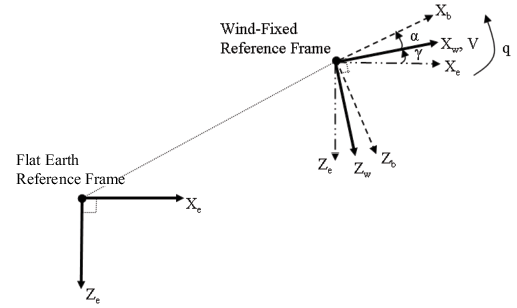Documentation

# Custom Variable Mass 3DOF (Wind Axes)

Implement three-degrees-of-freedom equations of motion of custom variable mass with respect to wind axes

## Library

Equations of Motion/3DOF## Description

The Custom Variable Mass 3DOF (Wind Axes) block considers the rotation in the vertical plane of a wind-fixed coordinate frame about a flat Earth reference frame.The equations of motion are

`$\begin{array}{l}\stackrel{˙}{V}=\frac{{F}_{{x}_{wind}}}{m}-\frac{\stackrel{˙}{m}Vr{e}_{{x}_{wind}}}{m}-g\mathrm{sin}\gamma \\ {A}_{be}=\left[\begin{array}{c}{A}_{{x}_{c}}\\ {A}_{{z}_{c}}\end{array}\right]=DC{M}_{wb}\left[\frac{{F}_{w}-\stackrel{˙}{m}{V}_{rew}}{m}-\overline{g}\right]\\ {A}_{bb}=\left[\begin{array}{c}{A}_{xb}\\ {A}_{zb}\end{array}\right]=DC{M}_{wb}\left[\frac{{F}_{w}-\stackrel{˙}{m}{V}_{rew}}{m}-g-{\overline{\omega }}_{w}×{\overline{V}}_{w}\right]\\ \stackrel{˙}{\alpha }=\frac{{F}_{{z}_{wind}}}{mV}+q+\frac{g}{V}\mathrm{cos}\gamma -\frac{\stackrel{˙}{m}Vr{e}_{{z}_{wind}}}{mV}\\ \stackrel{˙}{q}=\stackrel{˙}{\theta }=\frac{{M}_{{y}_{body}}-{\stackrel{˙}{I}}_{yy}q}{{I}_{yy}}\\ \stackrel{˙}{\gamma }=q-\stackrel{˙}{\alpha }\end{array}$`

where the applied forces are assumed to act at the center of gravity of the body. Vrew is the relative velocity in the wind axes at which the mass flow ($\stackrel{˙}{m}$) is ejected or added to the body in wind axes.

## Parameters

### Main

Units

Specifies the input and output units:

Units

Forces

Moment

Acceleration

Velocity

Position

Mass

Inertia

`Metric (MKS)`

Newton

Newton meter

Meters per second squared

Meters per second

Meters

Kilogram

Kilogram meter squared

```English (Velocity in ft/s)```

Pound

Foot pound

Feet per second squared

Feet per second

Feet

Slug

Slug foot squared

```English (Velocity in kts)```

Pound

Foot pound

Feet per second squared

Knots

Feet

Slug

Slug foot squared

Mass Type

Select the type of mass to use:

 `Fixed` Mass is constant throughout the simulation. ```Simple Variable``` Mass and inertia vary linearly as a function of mass rate. ```Custom Variable``` Mass and inertia variations are customizable.

The `Custom Variable` selection conforms to the previously described equations of motion.

Initial airspeed

A scalar value for the initial velocity of the body, (V0).

Initial flight path angle

A scalar value for the initial pitch attitude of the body, (γ0).

Initial incidence

A scalar value for the initial angle between the velocity vector and the body, (α0).

Initial body rotation rate

A scalar value for the initial body rotation rate, (q0).

Initial position (x,z)

A two-element vector containing the initial location of the body in the flat Earth reference frame.

Gravity Source

Specify source of gravity:

 `External` Variable gravity input to block `Internal` Constant gravity specified in Acceleration due to gravity
Acceleration due to gravity

A scalar value for the acceleration due to gravity used if internal gravity source is selected. If gravity is to be neglected in the simulation, this value can be set to `0`. This parameter appears if you set Gravity source to `Internal`.

Include mass flow relative velocity

Select this check box to add a mass flow relative velocity port. This is the relative velocity at which the mass is accreted or ablated.

Include inertial acceleration

Select this check box to enable an additional output port for the accelerations in body-fixed axes with respect to the inertial frame. You typically connect this signal to the accelerometer.

### State Attributes

Assign unique name to each state. You can use state names instead of block paths during linearization.

• To assign a name to a single state, enter a unique name between quotes, for example, `'velocity'`.

• To assign names to multiple states, enter a comma-delimited list surrounded by braces, for example, `{'a', 'b', 'c'}`. Each name must be unique.

• If a parameter is empty (`' '`), no name assignment occurs.

• The state names apply only to the selected block with the name parameter.

• The number of states must divide evenly among the number of state names.

• You can specify fewer names than states, but you cannot specify more names than states.

For example, you can specify two names in a system with four states. The first name applies to the first two states and the second name to the last two states.

• To assign state names with a variable in the MATLAB® workspace, enter the variable without quotes. A variable can be a character vector, cell array, or structure.

Velocity: e.g., 'V'

Specify velocity state name.

Default value is `''`.

Incidence angle: e.g., 'alpha'

Specify incidence angle state name.

Default value is `''`.

Flight path angle: e.g., 'gamma'

Specify flight path angle state name.

Default value is `''`.

Body rotation rate: e.g., 'q'

Specify body rotation rates state name.

Default value is `''`.

Position: e.g., {'Xe', 'Ze'}

Specify position state names.

Default value is `''`.

## Inputs and Outputs

InputDimension TypeDescription
First Contains the force acting along the wind x-axis, (Fx).
Second Contains the force acting along the wind z-axis, (Fz).
Third Contains the applied pitch moment in body axes, (M).
Fourth (Optional)VectorContains one or more rates of change of mass, $\left(\stackrel{˙}{m}\right)$ (positive if accreted, negative if ablated).
Fifth Contains the mass, (m).
Sixth Contains the rate of change of inertia tensor matrix, $\left({\stackrel{˙}{I}}_{yy}\right)$.
Seventh Contains the inertia tensor matrix, (Iyy).
Eighth (Optional) Contains the gravity in the selected units.

Ninth (Optional)

Two-element vectorContains one or more relative velocities at which the mass is accreted to or ablated from the body in wind axes.
OutputDimension TypeDescription
First Contains the flight path angle, within ±pi, in radians (γ).
Second Contains the pitch angular rate, in radians per second (ωy).
Third Contains the pitch angular acceleration, in radians per second squared (y/dt).
FourthTwo-element vectorContains the location of the body, in the flat Earth reference frame, (Xe, Ze).
FifthTwo-element vectorContains the velocity of the body resolved into the wind-fixed coordinate frame, (V, 0).
SixthTwo-element vectorContains the acceleration of the body resolved into the body-fixed coordinate frame, (Ax, Az).
SeventhScalarContains the angle of attack, (α).
Eight (Optional)Two-element vectorContains the accelerations in body-fixed axes with respect to inertial frame (flat Earth). You typically connect this signal to the accelerometer.

## Reference

Stevens, B. L., and F. L. Lewis, Aircraft Control and Simulation, John Wiley & Sons, New York, 1992.

#### Introduced in R2006a

##### Support평가판 신청